Next: Axisymmetric Stokes Flow Around Up: Incompressible Viscous Flow Previous: Stokes Flow

# Axisymmetric Stokes Flow

Let,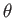,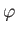be standard spherical coordinates. Consider axisymmetric Stokes flow such that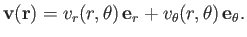(10.84)

According to Equations (A.175) and (A.176), we can automatically satisfy the incompressibility constraint (10.80) by writing (see Section 7.4)(10.85)

where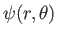is the Stokes stream function (i.e.,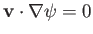). It follows that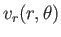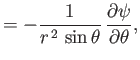(10.86)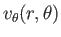(10.87)

Moreover, according to Section C.4,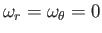, and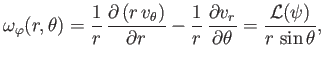(10.88)

where (see Section 7.4)(10.89)

Hence, given that, we can write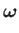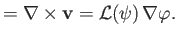(10.90)

It follows from Equations (A.176) and (A.178) that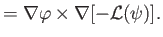(10.91)

Thus, by analogy with Equations (10.85) and (10.90), and making use of Equations (A.173) and (A.177), we obtain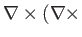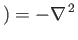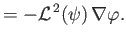(10.92)

Equation (10.83) implies that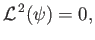(10.93)

which is the governing equation for axisymmetric Stokes flow. In addition, Equations (10.82) and (10.91) yield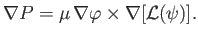(10.94)Next: Axisymmetric Stokes Flow Around Up: Incompressible Viscous Flow Previous: Stokes Flow
Richard Fitzpatrick 2016-03-31